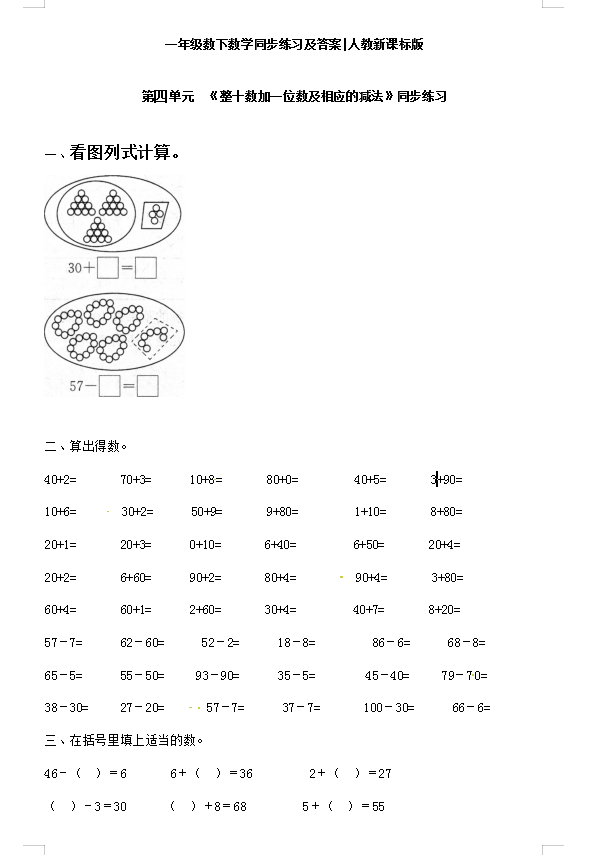【精品】小学一年级下册数学同步练习-《整十数加一位数及相应的减法》1-人教新课标（3页DOC文档）资料下载

40+2=       70+3=      10+8=       80+0=         40+5=       3+90=

10+6=       30+2=      50+9=       9+80=         1+10=       8+80=

20+1=       20+3=      0+10=       6+40=         6+50=       20+4=

20+2=       6+60=      90+2=       80+4=         90+4=       3+80=

60+4=       60+1=      2+60=       30+4=         40+7=       8+20=

57–7=      62–60=      52–2=      18–8=         86–6=      68–8=

65–5=      55–50=     93–90=      35–5=        45–40=     79–70=

38–30=     27–20=      57–7=      37–7=       100–30=      66–6